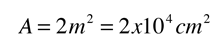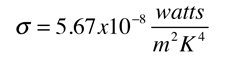The basic heat transfer equation for radiation iswhere A is the area of the human body and e is the emissivity of the skin. In this case the temperatures must be in Kelvins. A typical body area according to physiology texts:Human skin is a near-ideal radiator in the infrared. For a perfect radiator e=1.e = 0.97The Stefan-Boltzmann constant:Thot = 307K, Tcold = 296K.

Data may be entered for any of the parameters below, and the heat loss rate by radiation will be calculated. Default values will be entered for unspecified parameters, but all values may be changed.

For a skin area A = m2

and emissivity e = ,

 if the temperatures are Tskin = °C = K
 and Tambient = °C = K

then the heat loss rate will be

Q/t = watts.
 Modeling the cooling of the human body
Index

Heat transfer concepts

Heat transfer examples

 HyperPhysics***** Thermodynamics R Nave
Go Back